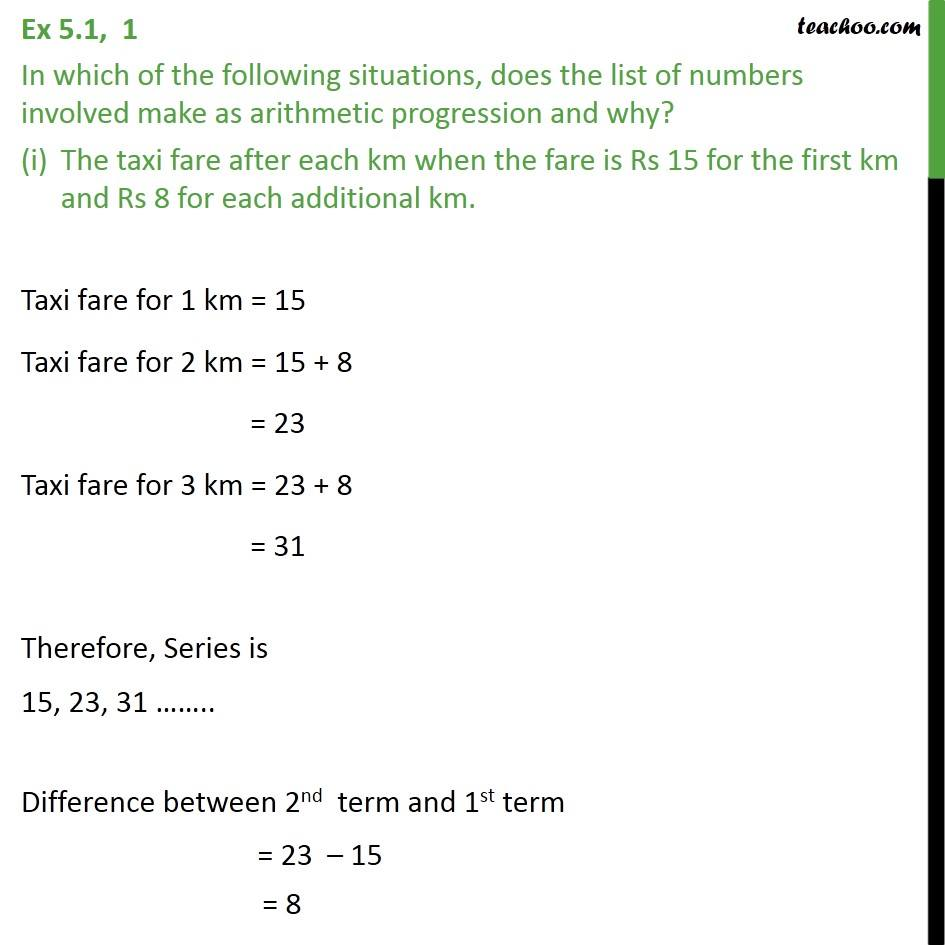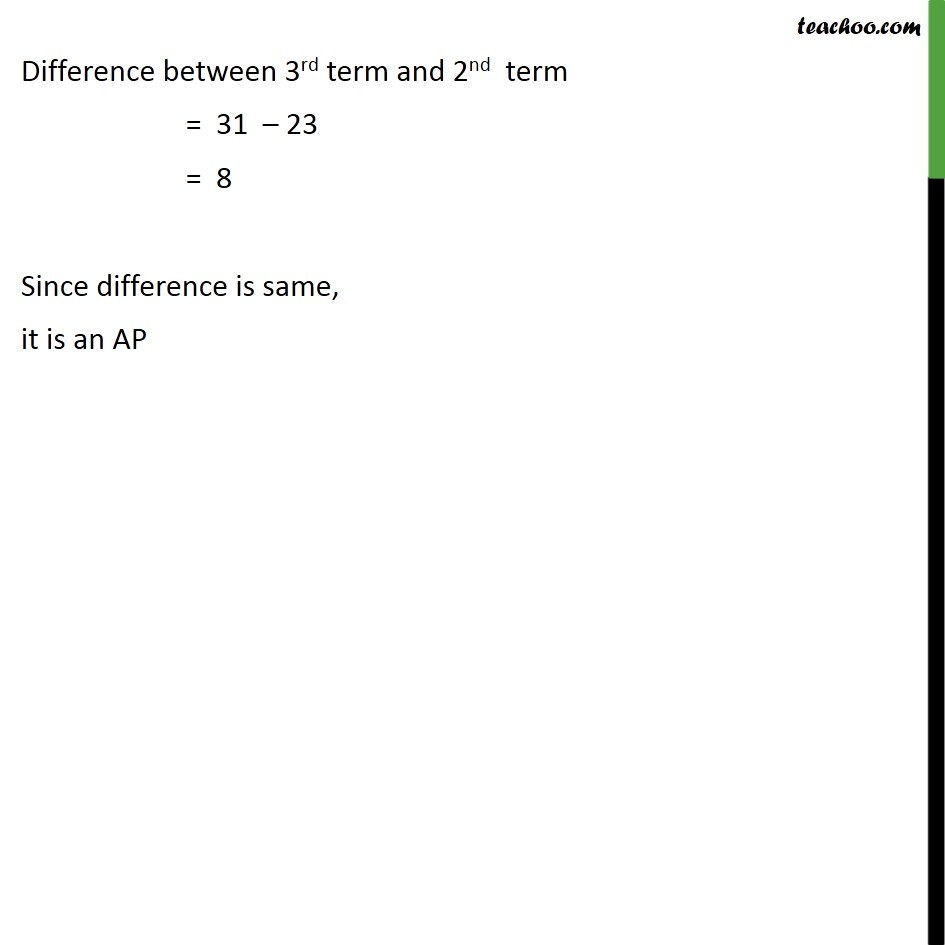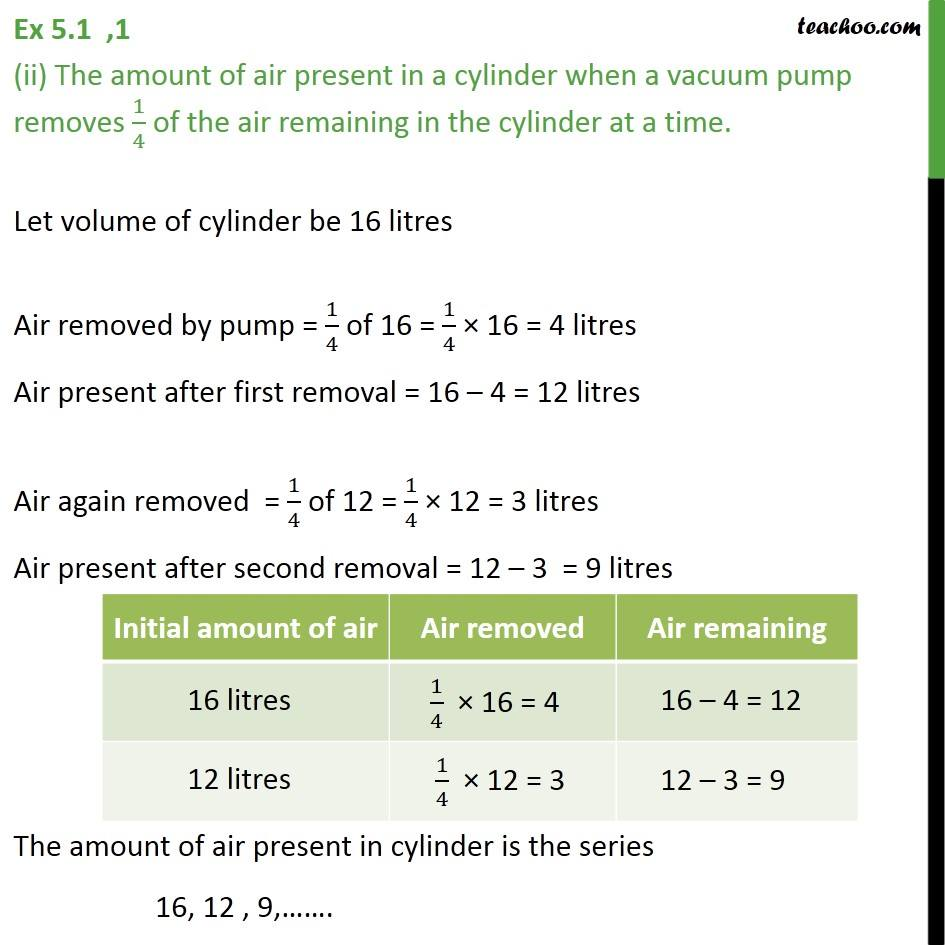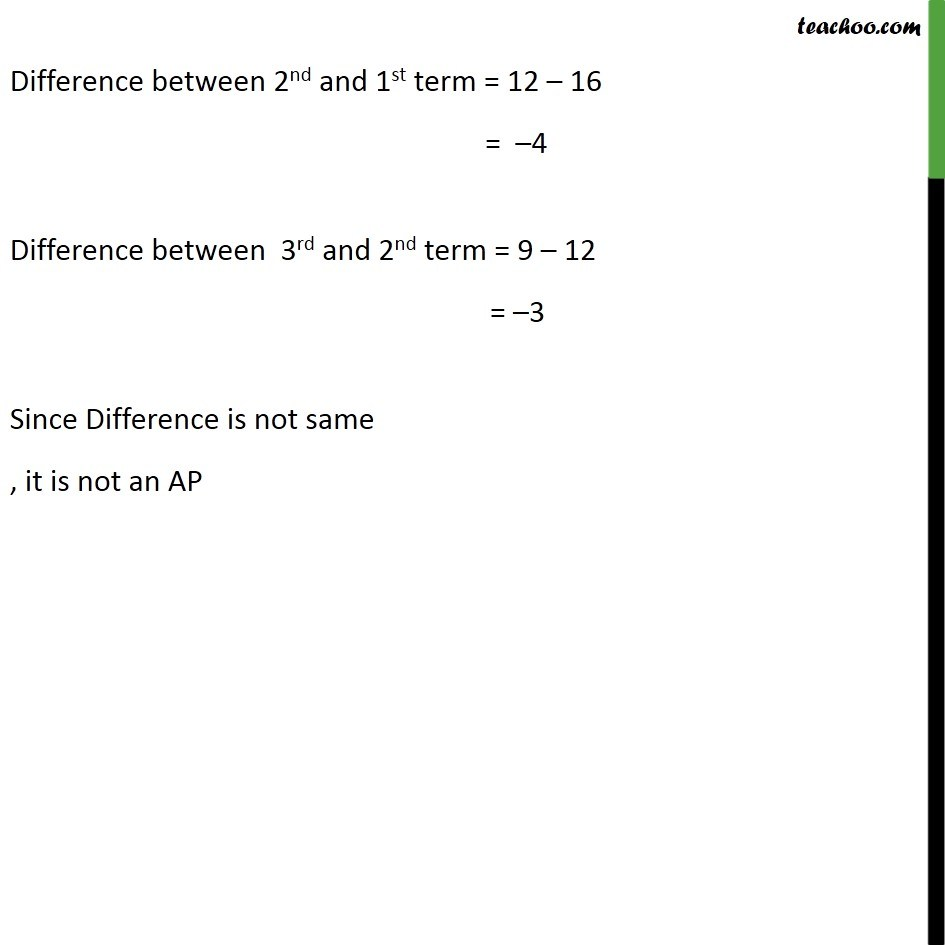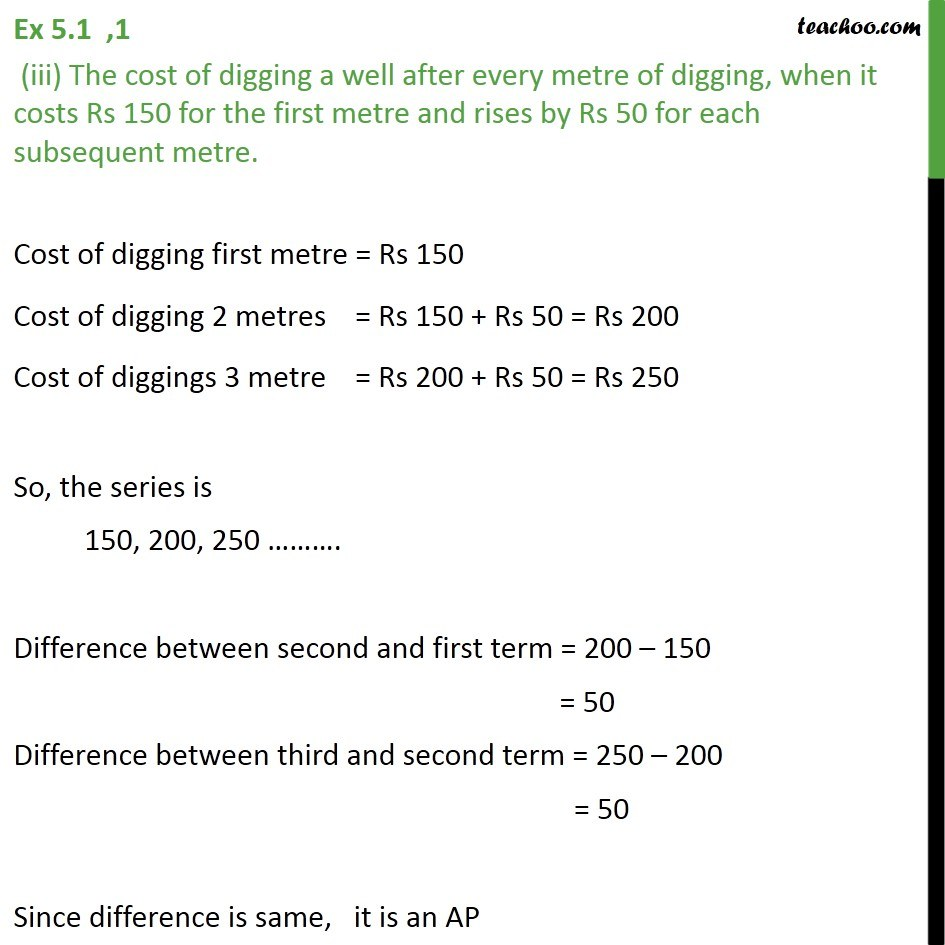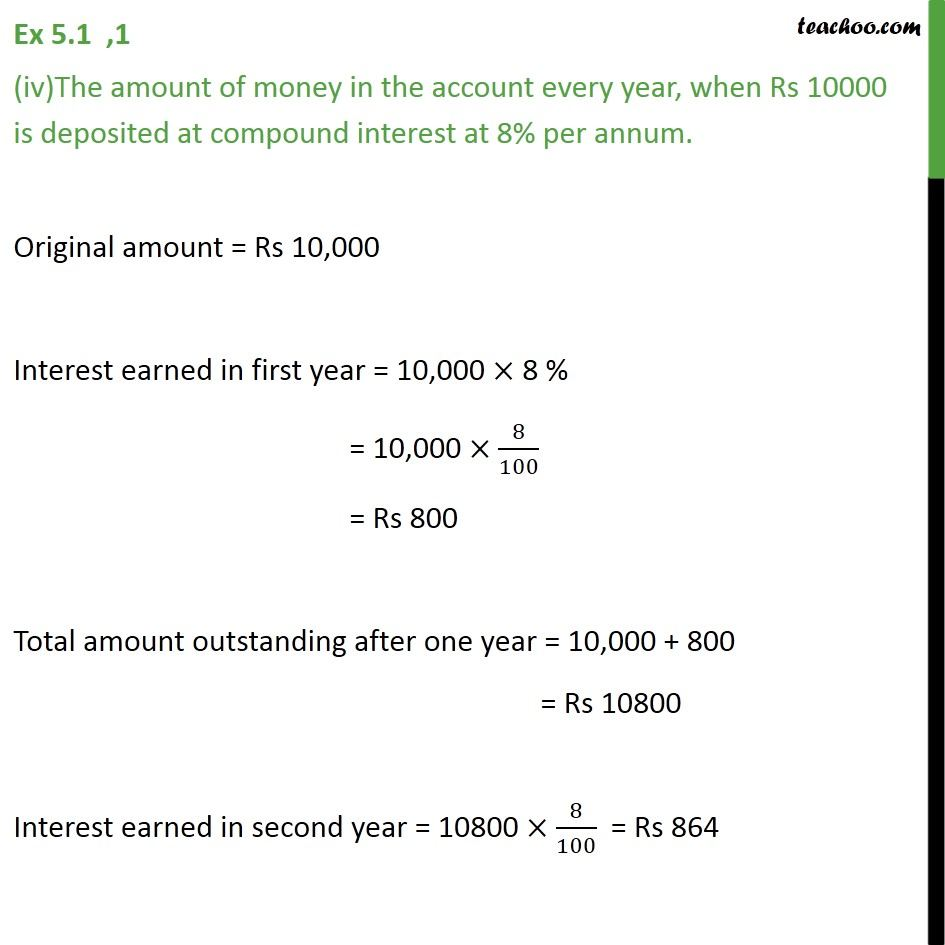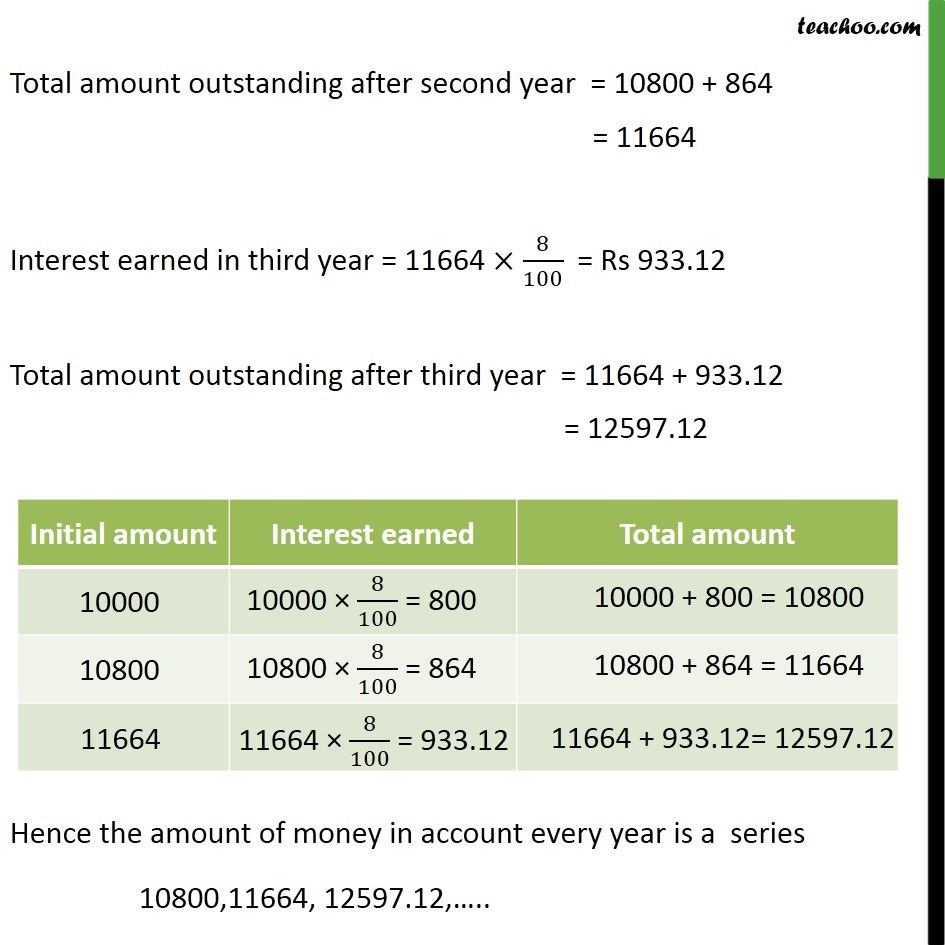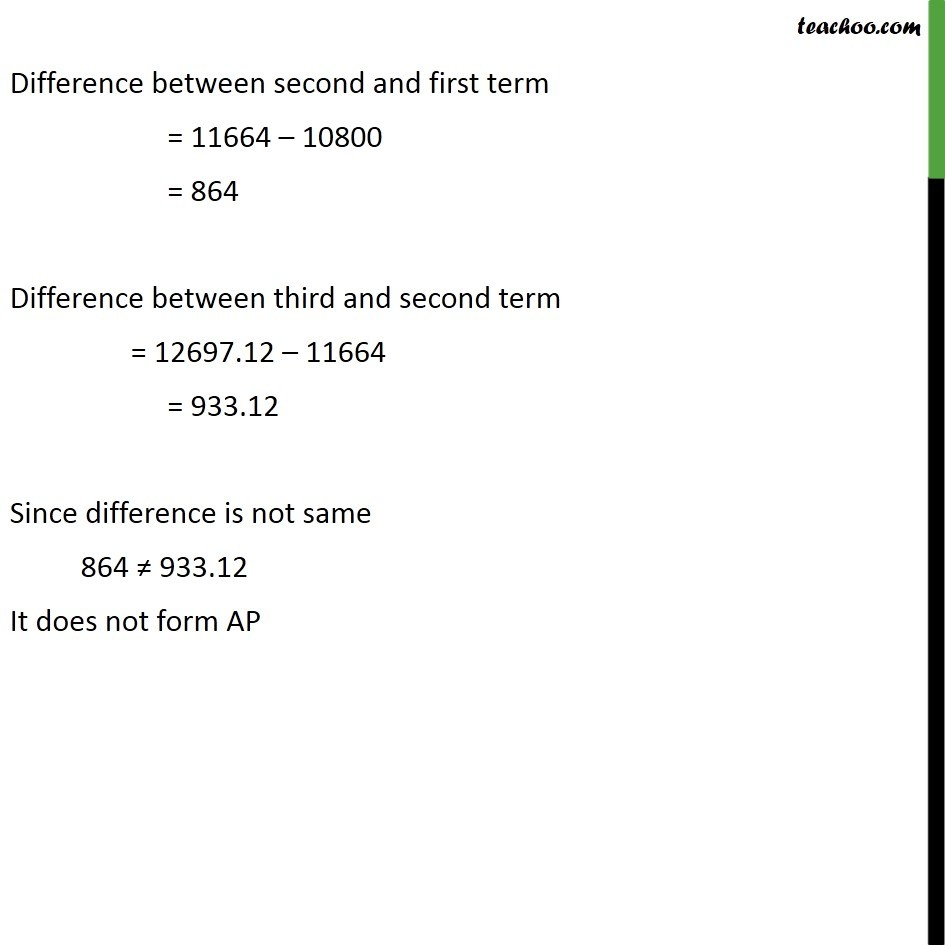1. Class 10
2. Important Questions for Exam - Class 10
3. Chapter 5 Class 10 Arithmetic Progressions

Transcript

Ex 5.1, 1 In which of the following situations, does the list of numbers involved make as arithmetic progression and why? The taxi fare after each km when the fare is Rs 15 for the first km and Rs 8 for each additional km. Taxi fare for 1 km = 15 Taxi fare for 2 km = 15 + 8 = 23 Taxi fare for 3 km = 23 + 8 = 31 Therefore, Series is 15, 23, 31 .. Difference between 2nd term and 1st term = 23 15 = 8 Difference between 3rd term and 2nd term = 31 23 = 8 Since difference is same, it is an AP Ex 5.1 ,1 (ii) The amount of air present in a cylinder when a vacuum pump removes 1/4 of the air remaining in the cylinder at a time. Let volume of cylinder be 16 litres Air removed by pump = 1/4 of 16 = 1/4 16 = 4 litres Air present after first removal = 16 4 = 12 litres Air again removed = 1/4 of 12 = 1/4 12 = 3 litres Air present after second removal = 12 3 = 9 litres The amount of air present in cylinder is the series 16, 12 , 9, . Difference between 2nd and 1st term = 12 16 = 4 Difference between 3rd and 2nd term = 9 12 = 3 Since Difference is not same , it is not an AP Ex 5.1 ,1 (iii) The cost of digging a well after every metre of digging, when it costs Rs 150 for the first metre and rises by Rs 50 for each subsequent metre. Cost of digging first metre = Rs 150 Cost of digging 2 metres = Rs 150 + Rs 50 = Rs 200 Cost of diggings 3 metre = Rs 200 + Rs 50 = Rs 250 So, the series is 150, 200, 250 . Difference between second and first term = 200 150 = 50 Difference between third and second term = 250 200 = 50 Since difference is same, it is an AP Ex 5.1 ,1 (iv)The amount of money in the account every year, when Rs 10000 is deposited at compound interest at 8% per annum. Original amount = Rs 10,000 Interest earned in first year = 10,000 8 % = 10,000 8/100 = Rs 800 Total amount outstanding after one year = 10,000 + 800 = Rs 10800 Interest earned in second year = 10800 8/100 = Rs 884 Total amount outstanding after second year = 10800 + 884 = 11664 Interest earned in third year = 11664 8/100 = Rs 933.12 Total amount outstanding after third year = 11664 + 933.12 = 12597.12 Hence the amount of money in account every year is a series 10800,11664, 12597.12, .. Difference between second and first term = 11664 10800 = 884 Difference between third and second term = 12697.12 11664 = 933.12 Since difference is not same 884 933.12 It does not form AP

Chapter 5 Class 10 Arithmetic Progressions

Class 10
Important Questions for Exam - Class 10